# P Block - Group 13 Questions and Answers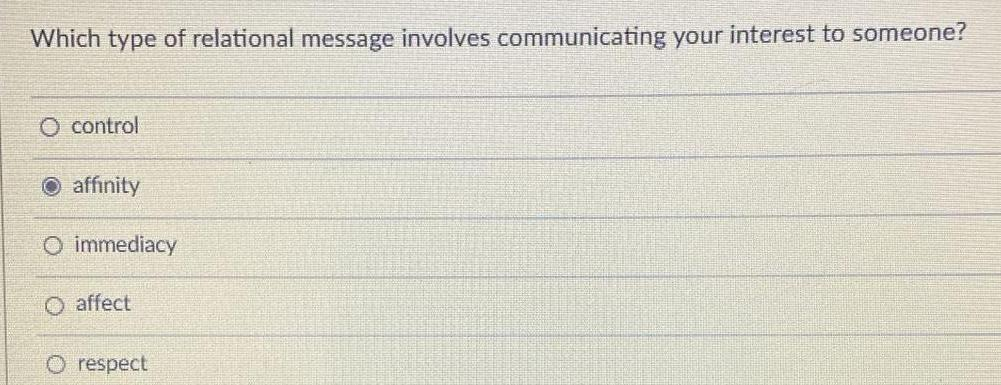Inorganic Chemistry
P Block - Group 13
Which type of relational message involves communicating your interest to someone? control affinity immediacy affect respect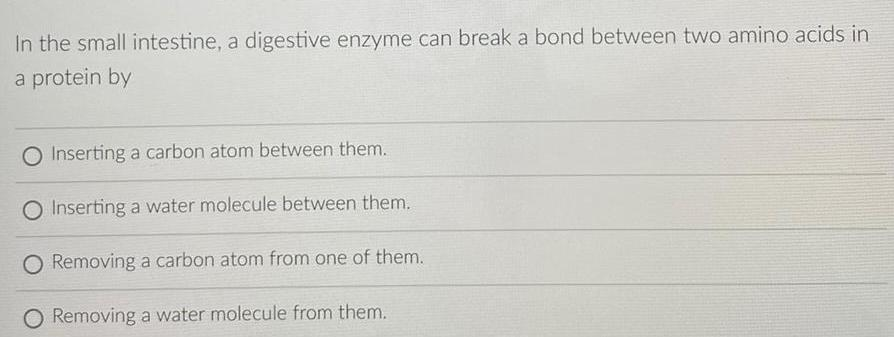Inorganic Chemistry
P Block - Group 13
In the small intestine, a digestive enzyme can break a bond between two amino acids in a protein by Inserting a carbon atom between them. Inserting a water molecule between them. Removing a carbon atom from one of them. Removing a water molecule from them.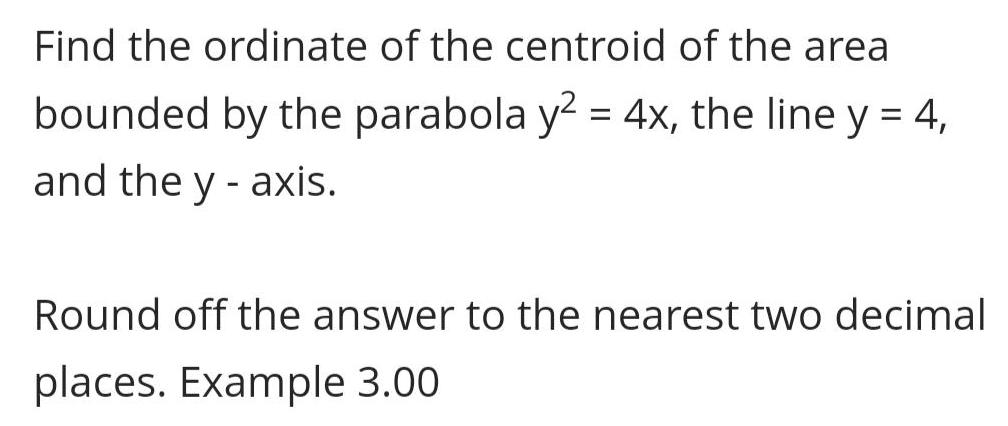Inorganic Chemistry
P Block - Group 13
Find the ordinate of the centroid of the area bounded by the parabola y² = 4x, the line y = 4, and the y -axis. Round off the answer to the nearest two decimal places.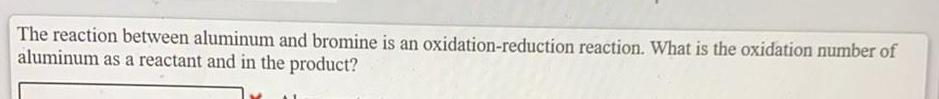Inorganic Chemistry
P Block - Group 13
The reaction between aluminum and bromine is an oxidation-reduction reaction. What is the oxidation number of aluminum as a reactant and in the product?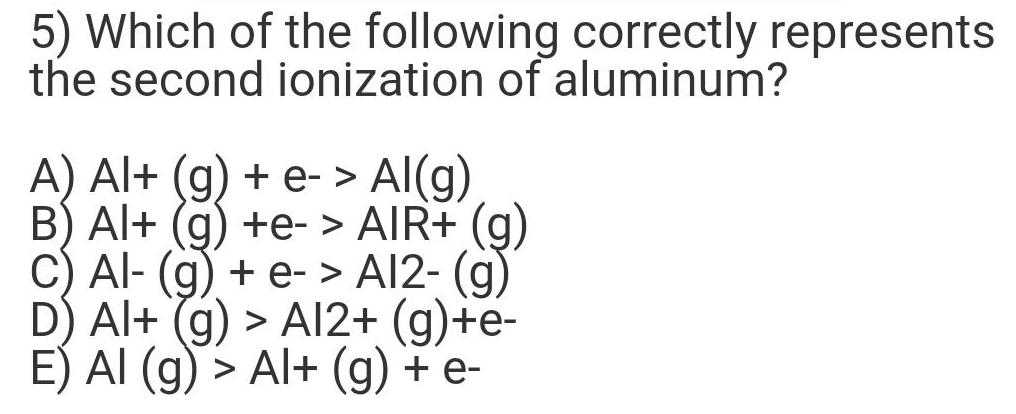Inorganic Chemistry
P Block - Group 13
Which of the following correctly represents the second ionization of aluminum? A) Al+ (g) + e-> Al(g) B) Al+ (g) +e-> AIR+ (g) C) Al- (g) + e- > A12- (g) D) Al+ (g) > A12+ (g)+e- E) AI (g) > Al+ (g) + e-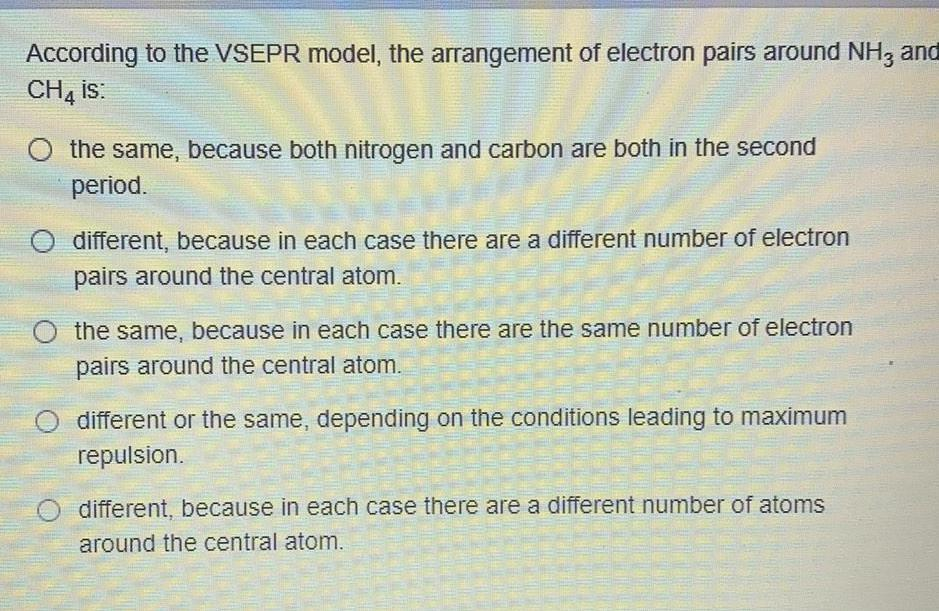Inorganic Chemistry
P Block - Group 13
According to the VSEPR model, the arrangement of electron pairs around NH3 and CH4 is: the same, because both nitrogen and carbon are both in the second period. different, because in each case there are a different number of electron pairs around the central atom. the same, because in each case there are the same number of electron pairs around the central atom. different or the same, depending on the conditions leading to maximum repulsion. different, because in each case there are a different number of atoms around the central atom.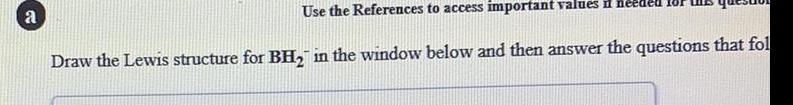Inorganic Chemistry
P Block - Group 13
Draw the Lewis structure for BH₂-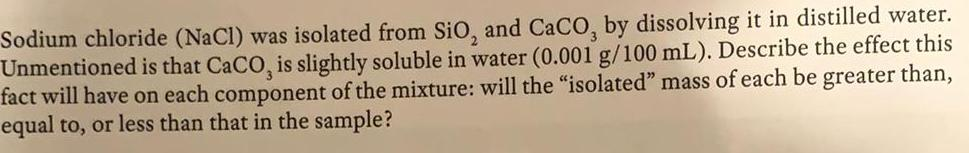Inorganic Chemistry
P Block - Group 13
Sodium chloride (NaCl) was isolated from SiO₂ and CaCO, by dissolving it in distilled water. Unmentioned is that CaCO, is slightly soluble in water (0.001 g/100 mL). Describe the effect this fact will have on each component of the mixture: will the "isolated" mass of each be greater than, equal to, or less than that in the sample?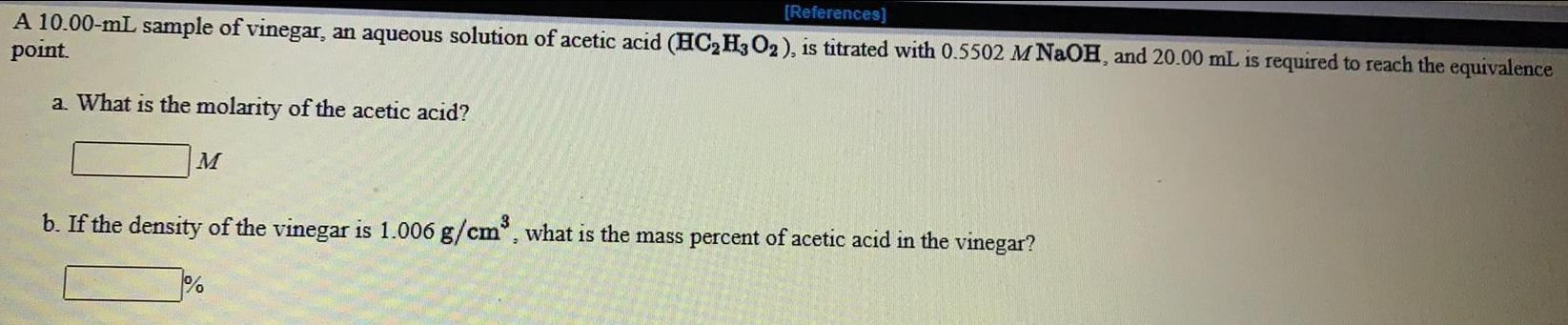Inorganic Chemistry
P Block - Group 13
A 10.00-mL sample of vinegar, an aqueous solution of acetic acid (HC₂H3O2), is titrated with 0.5502 M NaOH, and 20.00 mL is required to reach the equivalence point. a. What is the molarity of the acetic acid? b. If the density of the vinegar is 1.006 g/cm³, what is the mass percent of acetic acid in the vinegar?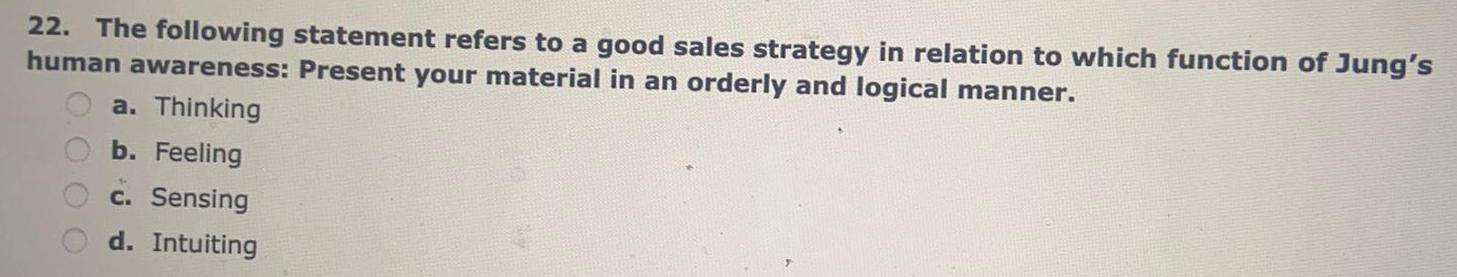Inorganic Chemistry
P Block - Group 13
The following statement refers to a good sales strategy in relation to which function of Jung's human awareness: Present your material in an orderly and logical manner. a. Thinking b. Feeling c. Sensing d. Intuiting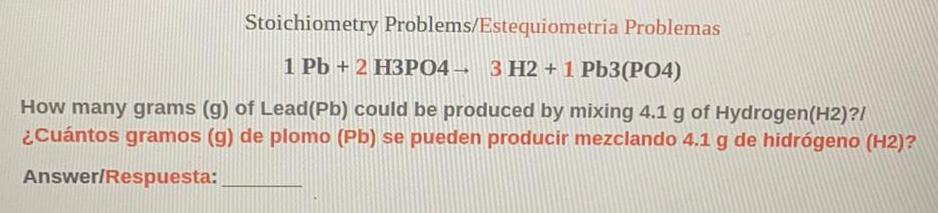Inorganic Chemistry
P Block - Group 13
Stoichiometry Problems/Estequiometria Problemas 1 Pb + 2 H3PO4 → 3 H2+1 Pb3(PO4) How many grams (g) of Lead (Pb) could be produced by mixing 4.1 g of Hydrogen (H2)?/ ¿Cuántos gramos (g) de plomo (Pb) se pueden producir mezclando 4.1 g de hidrógeno (H2)?Inorganic Chemistry
P Block - Group 13
2. Suggest a reason why it is desirable to use an excess of barium ion in the precipitation.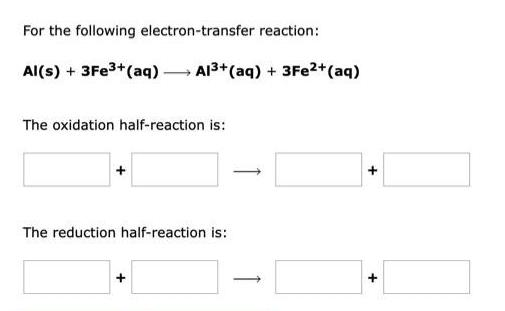Inorganic Chemistry
P Block - Group 13
For the following electron-transfer reaction: Al(s) + 3Fe3+ (aq) → Al3+ (aq) + 3Fe2+ (aq) The oxidation half-reaction is: The reduction half-reaction is: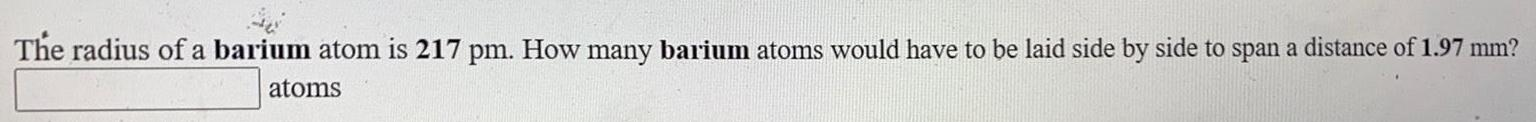Inorganic Chemistry
P Block - Group 13
The radius of a barium atom is 217 pm. How many barium atoms would have to be laid side by side to span a distance of 1.97 mm?
1